Solving problems by means of proportionCustom SearchSOLVING PROBLEMS BY MEANS OF PROPORTION One of the most common types of problems based on proportions involves triangles with proportional sides. Suppose that the corresponding sides of two triangles are known to be proportional. (See fig. 13-l.) The lengths of the sides of one triangle are 8, 9, and 11. The length of the side of the second triangle corresponding to side 8 in the first triangle is 10. We wish to find the lengths of the remaining sides, b and c.Figure 13- 1 .-Triangles with corresponding sides proportional. Since the corresponding sides are proportional, the pairs of corresponding sides may be used to form proportions as follows:To solve for b, we use the proportionand obtain the following result:The solution for c is similar to that for b, using the proportionwith the following result: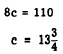The sides of the second triangle are 10, 11 1/4, and 13 3/4;. The result can also be obtained by using the factor of proportionality. Since 8 and 10 are lengths of corresponding sides, we can write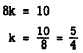The factor of proportionality is thus found to c be 5/4. Multiplying any side of the first triangle by 5/4 gives the corresponding side of the second triangle, as follows: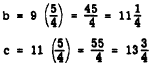Proportional sides of similar triangles may be used to determine the height of an object by measuring its shadow. (See fig. 13-2.)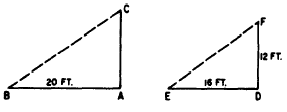Figure 13-2.-Measuring height by shadow length.  In figure 13-2, mast AC casts a shadow 20 ft. long (AB). At the same time, DF (12 ft. long) casts a shadow of 16 ft. long (DE). Assuming that both masts are vertical and on level ground, triangle ABC is similar to triangle DEF and their corresponding sides are therefore proportional. Thus the height of AC may be found as follows: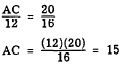Practice problems. In each of the following problems, set up a proportion and then solve for the unknown quantity: 1. Referring to figure 13-1, if the shortest side of the larger triangle is 16 units long, rather than 10, how long is side c? 2. If a mast 8 ft high casts a shadow 10 ft long, how high is a mast that casts a shadow 40 ft long? Answers:Integrated Publishing, Inc. - A (SDVOSB) Service Disabled Veteran Owned Small Business